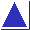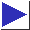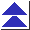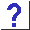# comp.lang.c FAQ list ·Question 20.7

Q: How can I manipulate individual bits?

A: Bit manipulation is straightforward in C, and commonly done. To extract (test) a bit, use the bitwise AND (&) operator, along with a bit mask representing the bit(s) you're interested in:

```	value & 0x04
```
To set a bit, use the bitwise OR (| or |=) operator:
```	value |= 0x04
```
To clear a bit, use the bitwise complement (~) and the AND (& or &=) operators:
```	value &= ~0x04
```
(The preceding three examples all manipulate the third-least significant, or 2**2, bit, expressed as the constant bitmask 0x04.)

To manipulate an arbitrary bit, use the shift-left operator (<<) to generate the mask you need:

```	value & (1 << bitnumber)
value |= (1 << bitnumber)
value &= ~(1 << bitnumber)
```
Alternatively, you may wish to precompute an array of masks:
```	unsigned int masks[] =
{0x01, 0x02, 0x04, 0x08, 0x10, 0x20, 0x40, 0x80};

```

To avoid surprises involving the sign bit, it is often a good idea to use unsigned integral types in code which manipulates bits and bytes.